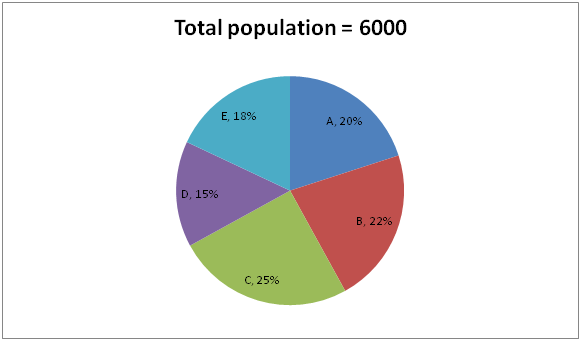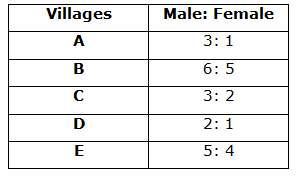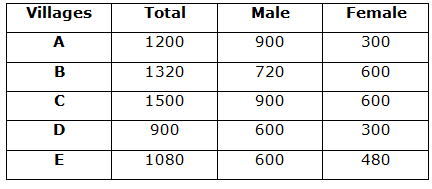# LIC Assistant Mains Quantitative Aptitude Questions 2019 – (Day-5)

Dear Aspirants, Our IBPS Guide team is providing new pattern Quantitative Aptitude Questions for LIC Assistant Mains 2019 so the aspirants can practice it on a daily basis. These questions are framed by our skilled experts after understanding your needs thoroughly. Aspirants can practice these new series questions daily to familiarize with the exact exam pattern and make your preparation effective.

Check here for LIC Assistant Mains Mock Test 2019

[WpProQuiz 7531]

Simplification

Directions (1 – 5): what value should come in place of question mark (?) in the following questions?

1) √3249 ÷ 3 + 3796416 = 93 + 12 % of 900 – ? × 5

a) 185.7

b) 216.2

c) 132.4

d) 254.6

e) None of these

2) 7 ¼ + 5 3/7 – 3 ½ – 4 2/7 = ? – 5 ¾

a) 10 9/14

b) 9 5/7

c) 11 4/5

d) 13 2/3

e) None of these

3) 5268024 – ? ÷ √1444 + (3/7) of 581 – 52 = 29

a) 15784

b) 18258

c) 21560

d) 12996

e) None of these

4) 7560 ÷ (36 % of 1750) = 98 × 6 5/7 of (? ÷ 14)

a) 15/23

b) 12/47

c) 21/36

d) 8/15

e) None of these

5) ? – 192 ÷ 475 × 1425 + 153 = 52 % of 6600 + (56784 ÷ 13)

a) 5508

b) 2456

c) 3274

d) 4520

e) None of these

Data Interpretation

Directions (6 – 10): Study the following information carefully and answer the questions given below.

The given pie chart shows the number of population in five different villages.The given table shows the ratio of the number of male to female population different villages.6) If the ratio of the number of male population to total number of literate population in A is 9: 8, then what is the difference between the illiterate population in village A and number of female in A?

a) 150

b) 100

c) 120

d) 140

e) 180

7) The number of female population in C is approximately what percent of the total population in E?

a) 52%

b) 54%

c) 56%

d) 50%

e) 48%

8) Which of the following village is number of male population is minimum?

a) C and D

b) Only B

c) A and C

d) D and E

e) Only A

9) What is the difference between the number of male and female population in all the cities together?

a) 1320

b) 1960

c) 1690

d) 1440

e) 1560

10) The number of female population E and A together is what percent of the total population in all the villages together?

a) 11%

b) 13%

c) 15%

d) 17%

e) 19%

Direction (1-5) :

(57/3) + 156 = 729 + (12/100)*900 – 5x

19 + 156 = 729 + 108 – 5x

5x = 729 + 108 – 19 -156

5x = 662

X = 132.4

7 ¼ + 5 3/7 – 3 ½ – 4 2/7 + 5 ¾ = x

X = (7 + 5 – 3 – 4 + 5) (1/4 + 3/7 – ½ – 2/7 + ¾)

X = 10 [(14 + 24 – 28 -16 + 42)/56]

X = 10 (36/56) = 10 (9/14)

174 – (x/38) + (3/7)*581 – 52 = 29

174 + 249 – 52 – 29 = (x/38)

342 = (x/38)

x = 38*342 = 12996

7560 ÷ [(36/100)*1750] = 98 *(47/7)*(x/14)

7560/630 = 47x

12 = 47x

X = 12/47

X – (19*19*1425)/475 + 3375 = (52/100)*6600 + (56784/13)

X – 1083 + 3375 = 3432 + 4368

X = 3432 + 4368 + 1083 – 3375

X = 5508

Direction (6 – 10):Number of literate population in A = 8/9 * 900 = 800

Illiterate population in A = 1200 – 800 = 400

Difference = 400 – 300 = 100

Required percentage = 600/1080 * 100 = 56%

Male population in city D and E has minimum.

Male = 900 + 720 + 900 + 600 + 600 = 3720

Female = 300 + 600 + 600 + 300 + 480 = 2280

Difference = 3720 – 2280 = 1440